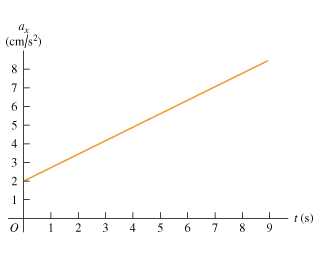Physics Practice Problems Velocity & Acceleration Graphs Practice Problems Solution: The graph in the figure describes the acceleration...

🤓 Based on our data, we think this question is relevant for Professor Karampagia's class at GVSU.

# Solution: The graph in the figure describes the acceleration as a function of time for a stone rolling down a hill starting from rest. (a) Find the change in the stones velocity between t = 2.5 and t = 7.5. (b) Sketch a graph of the stones velocity as a function of time.

###### Problem

The graph in the figure describes the acceleration as a function of time for a stone rolling down a hill starting from rest. (a) Find the change in the stones velocity between t = 2.5 and t = 7.5. (b) Sketch a graph of the stones velocity as a function of time.Velocity & Acceleration Graphs

Velocity & Acceleration Graphs

#### Q. The figure shows the position vs. time graph for two bicycles, A and B.Is there any instant at which the two bicycles have the same velocity?Which bic...

Solved • Mon Oct 29 2018 19:00:17 GMT-0400 (EDT)

Velocity & Acceleration Graphs

#### Q. The figure shows the velocity of a train as a function of time. (a) At what time was its velocity greatest?(b) During what periods, if any, was the ve...

Solved • Mon Oct 29 2018 19:00:11 GMT-0400 (EDT)

Velocity & Acceleration Graphs

#### Q. High-speed motion pictures ( 3500 ) of a jumping, 210 mu { m{g}} flea yielded the data used to plot the graph given in the figure . (See "The Flying L...

Solved • Mon Oct 29 2018 18:49:58 GMT-0400 (EDT)

Velocity & Acceleration Graphs

#### Q. A cat walks in a straight line, which we shall call the x-axis with the positive direction to the right. As an observant physicist, you make measureme...

Solved • Mon Oct 29 2018 18:49:57 GMT-0400 (EDT)